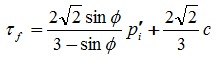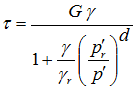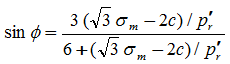# PressureIndependMultiYield Material

Jump to navigationJump to search

PressureIndependMultiYield material is an elastic-plastic material in which plasticity exhibits only in the deviatoric stress-strain response. The volumetric stress-strain response is linear-elastic and is independent of the deviatoric response. This material is implemented to simulate monotonic or cyclic response of materials whose shear behavior is insensitive to the confinement change. Such materials include, for example, organic soils or clay under fast (undrained) loading conditions.

During the application of gravity load (and static loads if any), material behavior is linear elastic. In the subsequent dynamic (fast) loading phase(s), the stress-strain response is elastic-plastic (see MATERIAL STAGE UPDATE below). Plasticity is formulated based on the multi-surface (nested surfaces) concept, with an associative flow rule. The yield surfaces are of the Von Mises type.

OUTPUT INTERFACE:

The following information may be extracted for this material at a given integration point, using the OpenSees Element Recorder facility (McKenna and Fenves 2001)®: "stress", "strain", "backbone", or "tangent".

For 2D problems, the stress output follows this order: σxx, σyy, σzz, σxy, ηr, where ηr is the ratio between the shear (deviatoric) stress and peak shear strength at the current confinement (0<=ηr<=1.0). The strain output follows this order: εxx, εyy, γxy.

For 3D problems, the stress output follows this order: σxx, σyy, σzz, σxy, σyz, σzx, ηr, and the strain output follows this order: εxx, εyy, εzz, γxy, γyz, γzx.

The "backbone" option records (secant) shear modulus reduction curves at one or more given confinements. The specific recorder command is as follows:

recorder Element –ele \$eleNum -file \$fName -dT \$deltaT material \$GaussNum backbone \$p1 <\$p2 …>

where p1, p2, … are the confinements at which modulus reduction curves are recorded. In the output file, corresponding to each given confinement there are two columns: shear strain γ and secant modulus Gs. The number of rows equals the number of yield surfaces.

 nDmaterial PressureIndependMultiYield \$tag \$nd \$rho \$refShearModul \$refBulkModul \$cohesi \$peakShearStra <\$frictionAng=0. \$refPress=100. \$pressDependCoe=0. \$noYieldSurf=20 <\$r1 \$Gs1 …> >

 \$tag A positive integer uniquely identifying the material among all nDMaterials. \$nd Number of dimensions, 2 for plane-strain, and 3 for 3D analysis. \$rho Saturated soil mass density. \$refShearModul (Gr) Reference low-strain shear modulus, specified at a reference mean effective confining pressure refPress of p’r (see below). \$refBulkModul (Br) Reference bulk modulus, specified at a reference mean effective confining pressure refPress of p’r (see below). \$cohesi (c) Apparent cohesion at zero effective confinement. \$peakShearStra (γmax) An octahedral shear strain at which the maximum shear strength is reached, specified at a reference mean effective confining pressure refPress of p’r (see below). \$frictionAng (Φ) Friction angle at peak shear strength in degrees, optional (default is 0.0). \$refPress (p’r) Reference mean effective confining pressure at which Gr, Br, and γmax are defined, optional (default is 100. kPa). \$pressDependCoe (d) A positive constant defining variations of G and B as a function of instantaneous effective confinement p’(default is 0.0):: If Φ=0, d is reset to 0.0. \$noYieldSurf Number of yield surfaces, optional (must be less than 40, default is 20). The surfaces are generated based on the hyperbolic relation defined in Note 2 below. \$r, \$Gs Instead of automatic surfaces generation (Note 2), you can define yield surfaces directly based on desired shear modulus reduction curve. To do so, add a minus sign in front of noYieldSurf, then provide noYieldSurf pairs of shear strain (γ) and modulus ratio (Gs) values. For example, to define 10 surfaces: … -10γ1Gs1 … γ10Gs10 … See Note 3 below for some important notes.

NOTE:

1. The friction angle Φ and cohesion c define the variation of peak (octahedral) shear strength τf as a function of current effective confinement p’i:2. Automatic surface generation: at a constant confinement p’, the shear stress τ(octahedral) - shear strain γ (octahedral) nonlinearity is defined by a hyperbolic curve (backbone curve):where γr satisfies the following equation at p’r:3. (User defined surfaces) The user specified friction angle Φ = 0. cohesion c will be ignored. Instead, c is defined by c=sqrt(3)*σm/2, where σm is the product of the last modulus and strain pair in the modulus reduction curve. Therefore, it is important to adjust the backbone curve so as to render an appropriate c.

If the user specifies Φ > 0, this Φ will be ignored. Instead, Φis defined as follows:If the resulting Φ <0, we set Φ=0 and c=sqrt(3)*σm/2.

Also remember that improper modulus reduction curves can result in strain softening response (negative tangent shear modulus), which is not allowed in the current model formulation. Finally, note that the backbone curve varies with confinement, although the variation is small within commonly interested confinement ranges. Backbone curves at different confinements can be obtained using the OpenSees element recorder facility (see OUTPUT INTERFACE above).

4. SUGGESTED PARAMETER VALUES

For user convenience, a table is provided below as a quick reference for selecting parameter values. However, use of this table should be of great caution, and other information should be incorporated wherever possible.

 Parameters Soft Clay Medium Clay Stiff Clay rho 1.3 ton/m3 or 1.217x10-4 (lbf)(s2)/in4 1.5 ton/m3 or 1.404x10-4 (lbf)(s2)/in4 1.8 ton/m3 or 1.685x10-4 (lbf)(s2)/in4 refShearModul 1.3x104 kPa or 1.885x103 psi 6.0x104 kPa or 8.702x104 psi 1.5x105 kPa or 2.176x104 psi refBulkModu 6.5x104 kPa or 9.427x103 psi 3.0x105 kPa or 4.351x104 psi 7.5x105 kPa or 1.088x105 psi cohesi 18 kPa or 2.611 psi 37 kPa or 5.366 psi 75 kPa or 10.878 psi peakShearStra (at p’r=80 kPa or 11.6 psi) 0.1 0.1 0.1 frictionAng 0 0 0 pressDependCoe 0 0 0

## Pressure Independent Material Examples:

 Material in elastic state Example 1 Single 2D plane-strain quadrilateral element, subjected to sinusoidal base shaking Example 2 Single 2D quadrilateral element, subjected to monotonic pushover (English units version)

Code Developed by: UC San Diego (Dr. Zhaohui Yang):

UC San Diego Soil Model:

• NDMaterial Command
• UC San Diego soil models (Linear/Nonlinear, dry/drained/undrained soil response under general 2D/3D static/cyclic loading conditions (please visit UCSD for examples)
• UC San Diego Saturated Undrained soil